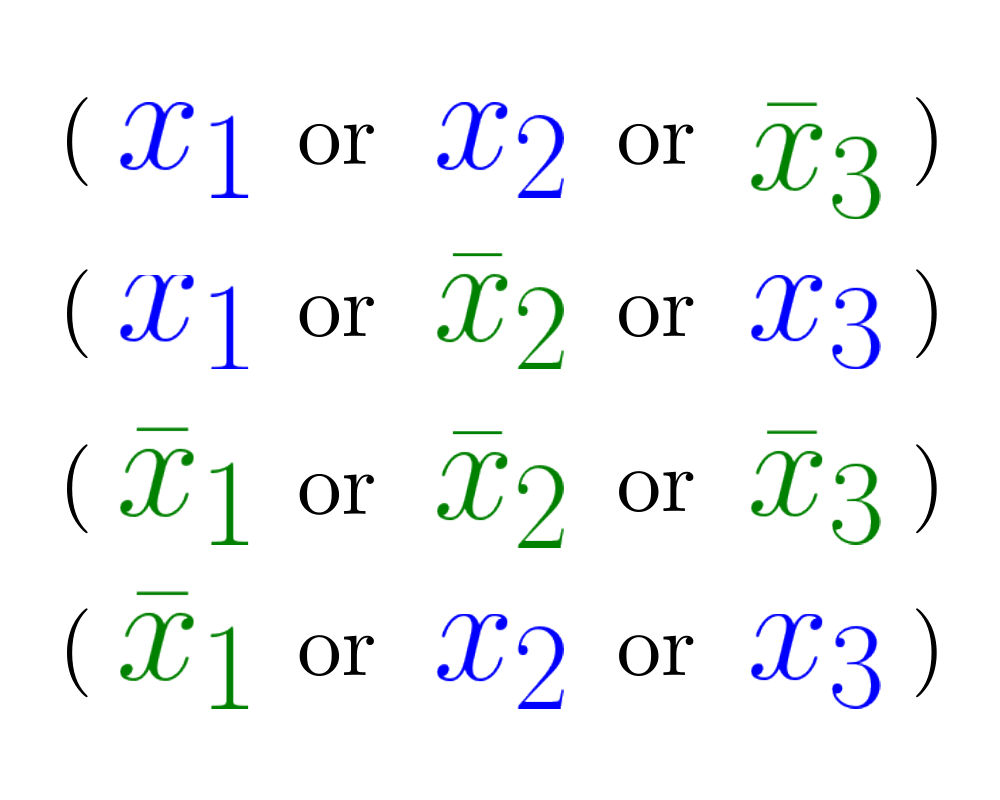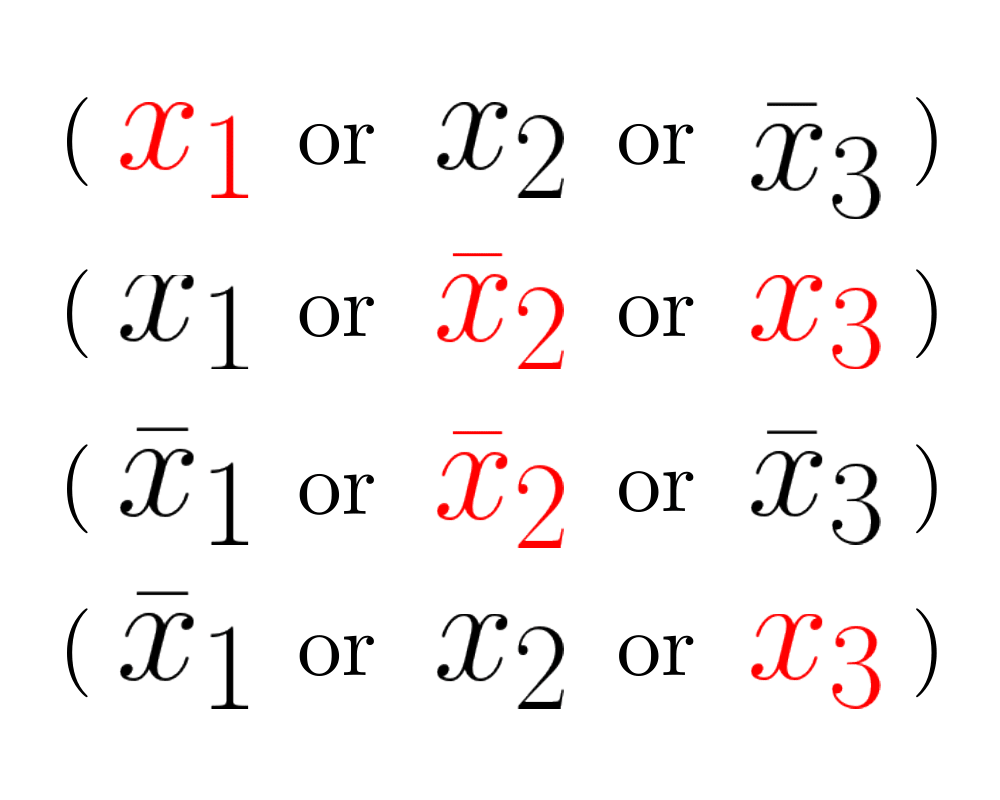# SatisfiabilityInputOutput

Input Description: A set of clauses in conjunctive normal form.
Problem: Is there a truth assignment to the boolean variables such that every clause is satisfied?

Excerpt from The Algorithm Design Manual: Satisfiability arises whenever we seek a configuration or object that must be consistent with (\ie satisfy) a given set of constraints. For example, consider the problem of drawing name labels for cities on a map. For the labels to be legible, we do not want the labels to overlap, but in a densely populated region many labels need to be drawn in a small space. How can we avoid collisions?

For each of the $$n$$ cities, suppose we identify two possible places to position its label, say right above or right below each city. We can represent this choice by a Boolean variable $$vi$$, which will be true if city $$ci$$'s label is above $$ci$$, otherwise $$vi = false$$. Certain pairs of labels may be forbidden, such as when $$ci$$'s above label would obscure $$cj$$'s below label. This pairing can be forbidden by the two-element clause $$(\bar{v_i} \OR v_j )$$, where $$\bar{v}$$ means not $$v$$''. Finding a satisfying truth assignment for the resulting set of clauses yields a mutually legible map labeling if one exists.

Satisfiability is the original NP-complete problem. Despite its applications to constraint satisfaction, logic, and automatic theorem proving, it is perhaps most important theoretically as the root problem from which all other NP-completeness proofs originate.

Go To Main Page